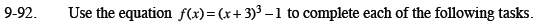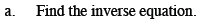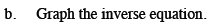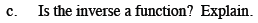Home > CCA2 > Chapter 9 > Lesson 9.3.2 > Problem9-92

9-92.
1. Use the equation f(x) = (x + 3)3 − 1 to complete each of the following tasks. 9-92 HW eTool (Desmos). Homework Help ✎

1. Find the inverse equation.

2. Graph the inverse equation.

3. Is the inverse a function? Explain.Solve for y: x = (y + 3)3 − 1How are a graph and its inverse graph related?
The graph of f(x) is given for you. Now sketch its inverse.Does the inverse graph pass the vertical line test?

Use the eTool below to help you with this problem.
Click on the link at right for the full eTool version: CCA2 9-92 HW eTool.